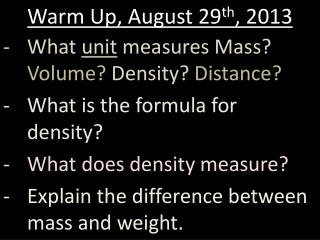DownloadDownload PresentationWarm Up, August 29 th , 2013

# Warm Up, August 29 th , 2013

Télécharger la présentation## Warm Up, August 29 th , 2013

- - - - - - - - - - - - - - - - - - - - - - - - - - - E N D - - - - - - - - - - - - - - - - - - - - - - - - - - -
##### Presentation Transcript

1. Warm Up, August 29th, 2013 • What unit measures Mass? Volume? Density? Distance? • What is the formula for density? • What does density measure? • Explain the difference between mass and weight.

2. Homework Check • Take out Volume, Area, Density Worksheet.

3. Homework Review Area = Length x Width 103 cm x 44 cm = 4532 cm 5 m x 2.5 m = 12.5 m

4. Homework Review Volume = Length x Width x Height (Depth) 4532 cm x 3 cm = 13596 cm 12.5 m x 1.2 m = 15 m

5. Homework Review Density = Mass / Volume 2000 g / 4000 cm3 = • ½ or 0.5 g/cm3 100 g / 125 mL = • 0.8 g/mL 400 g / 625 mL = • 0.64 g/mL

6. BrainPop: Measuring Matter

7. Area/Volume Lab • Groups of 3-4. • You will be given a #. That number is the number of your group. All students with the same number are in the same group. • Meet up as a group in four desks in the classroom, do not move desks.

8. Area/Volume Lab • Send one person to get the following: • Meter Stick • Lab Sheet • Calculator

9. Area/Volume Lab • Complete the questions on the lab sheet for a lab grade. • Use of correct units on the back and math work are required.